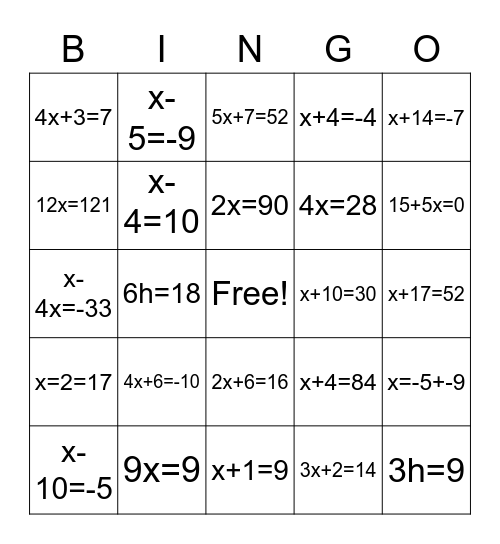# Math Bingo!This bingo card has a free space and 29 words: x-10=-5, 2x-3=15, 6h=18, 2x-10=6, 4x+3=7, 5x+7=52, x-4=10, 4x+6=-10, x+10=30, 9x=9, x+17=52, 3h=9, x+1=9, 3x+2=14, 15+5x=0, 2y+12=80, 2x+6=16, x=2=17, x+14=-7, x-4x=-33, x+4=84, x-4=18, 4x=28, 12x=121, x+4=-4, x-5=-9, x=-5+-9, 2x=90 and x=-2+-3.

## Play Online